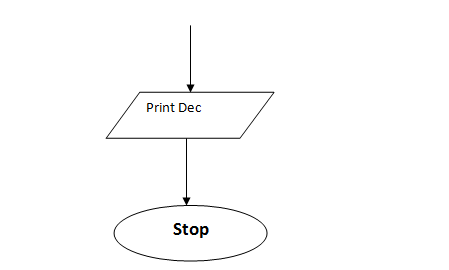# Draw a flow chart and write its corresponding C program to convert an octal number to its equivalent decimal number.

IGNOU Assignment Answers and free solutionsCategory: Assignment SolutionsDraw a flow chart and write its corresponding C program to convert an octal number to its equivalent decimal number.

Draw a flow chart and write its corresponding C program to convert an octal number to its equivalent decimal number.
Course Code: MCS-011
Course Title: Problem Solving and Programming

Octal is a numbering system that uses eight digits, 0 to 7, arranged in a series of columns to
represent all numerical quantities. Each column or place value has a weighted value of 1, 8, 64, 512,
and so on, ranging from right to left. Decimal is a term that describes the base-10 number system
commonly used by lay people in the developed world.Below is the Program in C:
/*
* C Program to Convert Octal to Decimal
*/
#include <stdio.h>
#include <math.h>
int main()
{
long int octal, decimal = 0;
int i = 0;
printf(“Enter any octal number: “);
scanf(“%ld”, &octal);
while (octal != 0)
{
decimal = decimal +(octal % 10)* pow(8, i++);
octal = octal / 10;
}
printf(“Equivalent decimal value: %ld”,decimal);
return 0;
}
Output:
Enter any octal number: 67
Equivalent decimal value: 55